# Review — Unsupervised Feature Learning via Non-Parametric Instance Discrimination

## Outperforms SOTA such as Context Prediction, Colorization, Jigsaw Puzzles, Split-Brain Auto, & Adversarial (BiGAN)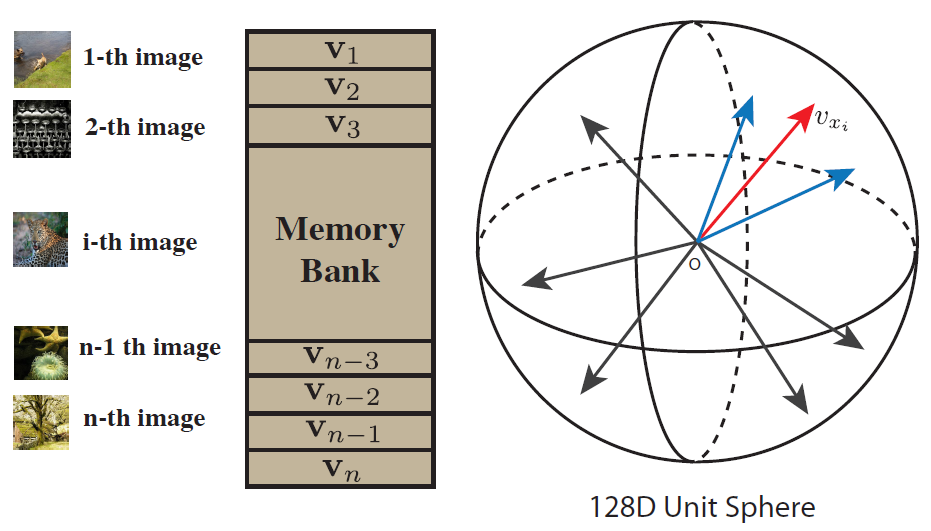Each image is treated as a class and projected to hypersphere

Unsupervised Feature Learning via Non-Parametric Instance Discrimination
Instance Discrimination
, by UC Berkeley / ICSI, Chinese University of Hong Kong, and Amazon Rekognition
2018 CVPR, Over 1100 Citations (Sik-Ho Tsang @ Medium)
Unsupervised Learning, Deep Metric Learning, Self-Supervised Learning, Semi-Supervised Learning, Image Classification, Object Detection

• Authors start by asking a question: “Can we learn a good feature representation that captures apparent similarity among instances, instead of classes, by merely asking the feature to be discriminative of individual instances?”
• This intuition is formulated as a non-parametric classification problem at the instance-level, and use noise-contrastive estimation (NCE) to tackle the computational challenges imposed by the large number of instance classes.

# Outline

1. Feature Learning via Non-Parametric Instance Discrimination
2. Learning with A Memory Bank and NCE
3. Proximal Regularization
4. Weighted k-Nearest Neighbor Classifier
5. Experimental Results

# 1. Unsupervised Feature Learning via Non-Parametric Instance Discrimination

## 1.1. Goal

• A backbone CNN is used to encode each image as a feature vector, which is projected to a 128-dimensional space and L2 normalized.
• The optimal feature embedding is learned via instance-level discrimination, which tries to maximally scatter the features of training samples over the 128-dimensional unit sphere.
• The goal is to learn an embedding function v=(x) without supervision. is a deep neural network with parameters θ, mapping image x to feature v.
• A metric is induced over the image space for instances x and y:

A good embedding should map visually similar images closer to each other.

• Each image instance is treated as a distinct class of its own and a classifier is trained to distinguish between individual instance classes.

## 1.2. Parametric Classifier: Conventional Softmax

• If we got n images/instances, we got n classes.
• Under the conventional parametric softmax formulation, for image x with feature v=(x), the probability of it being recognized as i-th example is:
• where wj is a weight vector for class j, and wTj v measures how well v matches the j-th class i.e., instance.

## 1.3. Proposed Non-Parametric Softmax Classifier

• A non-parametric variant of the above softmax equation is to replace wTj v with vTj v, and ||v|| = 1 is enforced via a L2-normalization layer.
• Then the probability P(i|v) becomes:
• where τ is a temperature parameter that controls the concentration level of the distribution (Please feel free to read Distillation for more details about temperature τ.) τ is important for supervised feature learning , and also necessary for tuning the concentration of v on the unit sphere.
• The learning objective is then to maximize the joint probability:
• or equivalently to minimize the negative log-likelihood over the training set:

Getting rid of these weight vectors is important, because the learning objective focuses entirely on the feature representation and its induced metric, which can be applied everywhere in the space and to any new instances at the test time.

• Also, it eliminates the need for computing and storing the gradients for {wj}, making it more scalable for big data applications.

# 2. Learning with A Memory Bank and NCE

## 2.1. Memory Bank

• To compute the probability P(i|v), {vj} for all the images are needed.
• Instead of exhaustively computing these representations every time, a feature memory bank V is maintained for storing them.
• Separate notations are introduced for the memory bank and features forwarded from the network. Let V = {vj} be the memory bank and fi=(xi) be the feature of xi.
• During each learning iteration, the representation fi as well as the network parameters θ are optimized via stochastic gradient descend.
• Then fi is updated to V at the corresponding instance entry fivi.
• All the representations in the memory bank V are initialized as unit random vectors.

## 2.2. Noise-Contrastive Estimation (NCE)

• Noise-Contrastive Estimation (NCE) is used to approximate full Softmax.

The basic idea is to cast the multi-class classification problem into a set of binary classification problems, where the binary classification task is to discriminate between data samples and noise samples.

• (NCE is originally used in NLP. Please feel free to read NCE if interested.)
• Specifically, the probability that feature representation v in the memory bank corresponds to the i-th example under the model is:
• where Zi is the normalizing constant. The noise distribution is formalized as a uniform distribution: Pn=1/n.
• Noise samples are assumed to be m times more frequent than data samples. The posterior probability of sample i with feature v being from the data distribution (denoted by D=1) is:
• The approximated training objective is to minimize the negative log-posterior distribution of data and noise samples:
• Here, Pd denotes the actual data distribution. For Pd, v is the feature corresponding to xi; whereas for Pn, v' is the feature from another image, randomly sampled according to noise distribution Pn.
• Both v and v’ are sampled from the non-parametric memory bank V.
• Computing normalizing constant Zi is expensive, Morte Carlo approximation is used:
• where {jk} is a random subset of indices. Empirically, the approximation derived from initial batches is sufficient to work well in practice.
• NCE reduces the computational complexity from O(n) to O(1) per sample.

# 3. Proximal Regularization

• During each training epoch, each class is only visited once. Therefore, the learning process oscillates a lot from random sampling fluctuation.

• At current iteration t, the feature representation for data xi is computed from the network v(t)i = (xi). The memory bank of all the representation are stored at previous iteration V={v(t-1)}.
• The loss function for a positive sample from Pd is:
• As learning converges, the difference between iterations, i.e. v(t)i- v(t-1)i, gradually vanishes, and the augmented loss is reduced to the original one.
• With proximal regularization, the final objective becomes:

The above figure shows that, empirically, proximal regularization helps stabilize training, speed up convergence, and improve the learned representation, with negligible extra cost.

# 4. Weighted k-Nearest Neighbor Classifier

• To classify test image ^x, we first compute its feature ^f=(^x), and then compare it against the embeddings of all the images in the memory bank, using the cosine similarity si=cos(vi, ^f).
• The top k nearest neighbors, denoted by Nk, would then be used to make the prediction via weighted voting.
• τ=0.07 and k=200.

# 5. Experimental Results

## 5.1. Parametric vs. Non-Parametric Softmax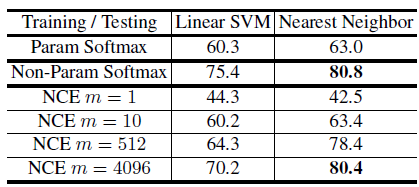Top-1 accuracies on CIFAR10, by applying linear SVM or kNN classifiers on the learned features.
• ResNet-18 is used as the backbone network and its output features mapped into 128-dimensional vectors.
• A common practice is to train an SVM on the learned feature over the training set, and to then classify test instances based on the feature extracted from the trained network. In addition, nearest neighbor classifiers are also used to assess the learned feature.
• With parametric softmax, accuracies of 60.3% and 63.0% with linear SVM and kNN classifiers respectively.

With non-parametric softmax, the accuracy rises to 75.4% and 80.8% for the linear SVM and nearest neighbour classifiers, a remarkable 18% boost for the latter.

• The NCE approximation is controlled by m, the number of negatives drawn for each instance.
• With m=1, the accuracy with kNN drops significantly to 42.5%.
• As m increases, the performance improves steadily. When m=4,096, the accuracy approaches that at m=49,999 — full form evaluation without any approximation.
• This result provides assurance that NCE is an efficient approximation.

## 5.2. Image Classification

• Linear SVM is trained on the intermediate features from conv1 to conv5. Note that there are also corresponding layers in VGG16 and ResNet.
• kNN is performed on the output features.

With AlexNet and linear classification on intermediate features, the proposed method achieves an accuracy of 35.6%, outperforming all baselines, including the state-of-the-art such as Context Prediction, Colorization, Jigsaw Puzzles, Split-Brain Auto, and Adversarial (BiGAN).

• The proposed method can readily scale up to deeper networks.
• As we move from AlexNet to ResNet-50, the accuracy is raised to 42.5%, whereas the accuracy with Exemplar-CNN is only 31.5% even with ResNet-101.
• Using nearest neighbor classification on the final 128 dimensional features, the proposed method achieves 31.3%, 33.9%, 40.5% and 42.5% accuracies with AlexNet, VGG16, ResNet-18 and ResNet-50, not much lower than the linear classification results.
• For Split-Brain Auto, the accuracy drops to 8.9% with nearest neighbor classification on conv3 features, and to 11.8% after projecting the features to 128 dimensions.

With the proposed method, the performance gradually increases for later layers. With all other methods, the performance decreases beyond conv3 or conv4.

• The intermediate layers can have over 10000 dimensions. The proposed method produces a 128-dimensional representation at the last layer, which is very efficient to work with.
• The encoded features of all 1.28M images in ImageNet only take about 600 MB of storage. Exhaustive nearest neighbor search over this dataset only takes 20 ms per image on a Titan X GPU.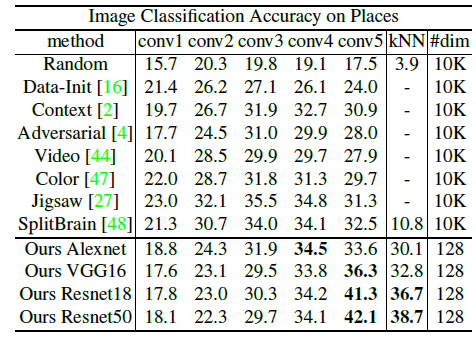Top-1 classification accuracies on Places, based on features learned on ImageNet, without fine-tuning
• The feature extraction networks trained on ImageNet are directly used for Place dataset without finetuning.
• Again, with linear classifier on conv5 features, the proposed method achieves competitive performance of top-1 accuracy 34.5% with AlexNet, and 42.1% with ResNet-50.
• With nearest neighbors on the last layer which is much smaller than intermediate layers, an accuracy of 38.7% is achieved with ResNet-50.
• These results show remarkable generalization ability of the representations learned using the proposed method.
• The testing accuracy continues to improve as training proceeds, with no sign of overfitting.
• The embedding size is varied from 32 to 256.
• The performance increases from 32, plateaus at 128, and appears to saturate towards 256.
• The feature learning method benefits from larger training sets, and the testing accuracy improves as the training set grows.
• This property is crucial for successful unsupervised learning, as there is no shortage of unlabeled data in the wild.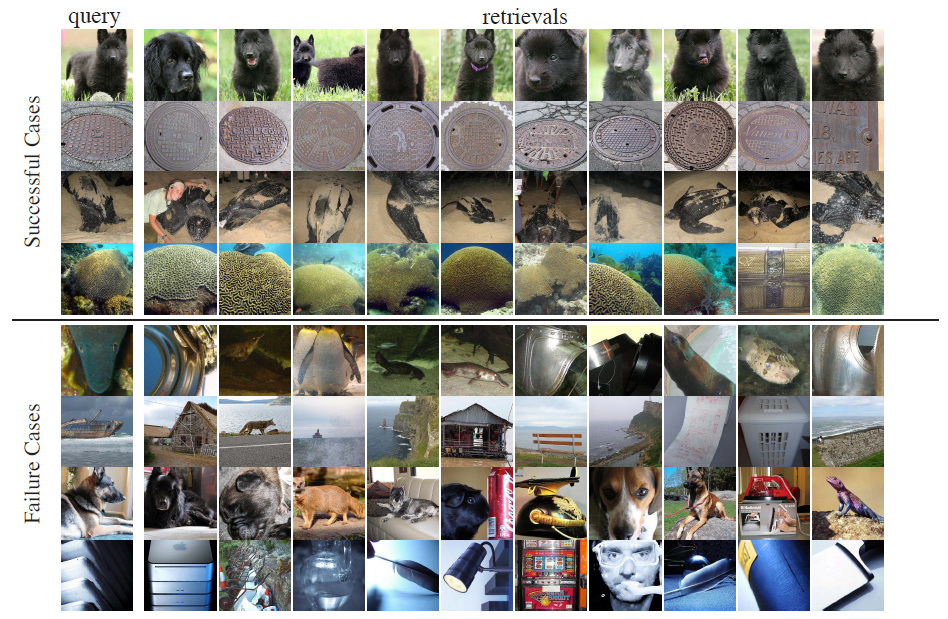Retrieval results for example queries. The left column are queries from the validation set, while the right columns show the 10 closest instances from the training set.
• Even for the failure cases, the retrieved images are still visually similar to the queries, a testament to the power of the proposed unsupervised learning objective.

## 5.3. Semi-Supervised Learning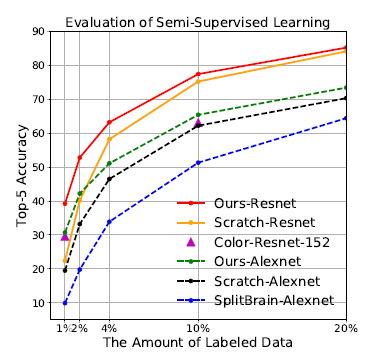Semi-supervised learning results on ImageNet with an increasing fraction of labeled data (x axis).
• A common scenario that can benefit from unsupervised learning is when we have a large amount of data of which only a small fraction are labeled.
• A natural semi-supervised learning approach is to first learn from the big unlabeled data and then fine-tune the model on the small labeled data.
• The proportion of labeled subset is varied from 1% to 20% of the entire dataset.

When only 1% of data is labeled, the proposed approach outperforms by a large 10% margin, demonstrating that the proposed feature learned from unlabeled data is effective for task adaptation.

## 5.4. Object Detection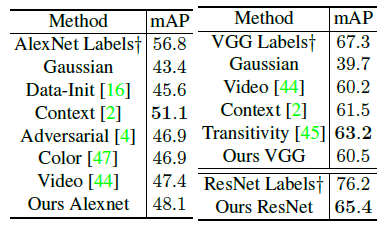Object detection performance on PASCAL VOC 2007 test, in terms of mean average precision (mAP)

With AlexNet/VGG16, the proposed method achieves an mAP of 48.1% and 60.5%, on par with the state-of-the-art unsupervised methods.

With ResNet-50, the proposed method achieves an mAP of 65.4%, surpassing all existing unsupervised learning approaches.

• It also shows that the proposed method scales well as the network gets deeper. There remains a significant gap of 11% to be narrowed towards mAP 76.2% from supervised pretraining.

This is one important literature before reading other self-supervised learning such as MoCo and CMC.

## Reference

[2018 CVPR] [Instance Discrimination]
Unsupervised Feature Learning via Non-Parametric Instance Discrimination

## Unsupervised/Self-Supervised Learning

2008–2010 [Stacked Denoising Autoencoders] 2014 [Exemplar-CNN] 2015 [Context Prediction] [Wang ICCV’15] 2016 [Context Encoders] [Colorization] [Jigsaw Puzzles] 2017 [L³-Net] [Split-Brain Auto] [Motion Masks] [Doersch ICCV’17] 2018 [RotNet/Image Rotations] [DeepCluster] [CPC/CPCv1] [Instance Discrimination]

## More from Sik-Ho Tsang

PhD, Researcher. I share what I've learnt and done. :) My LinkedIn: https://www.linkedin.com/in/sh-tsang/, My Paper Reading List: https://bit.ly/33TDhxG

Love podcasts or audiobooks? Learn on the go with our new app.

## The Story of Machine Learning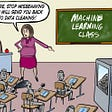## Machine Learning In a Nutshell## 3D Object Detection on LiDAR Point Clouds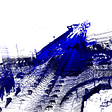## Build a Recommender System in less than an hour using Amazon Personalize## Understanding SSD MultiBox — Real-Time Object Detection In Deep Learning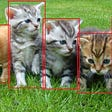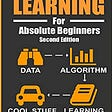## Sik-Ho Tsang

PhD, Researcher. I share what I've learnt and done. :) My LinkedIn: https://www.linkedin.com/in/sh-tsang/, My Paper Reading List: https://bit.ly/33TDhxG

## Review — Big Transfer (BiT): General Visual Representation Learning## Review — Vision Transformer with Deformable Attention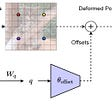## Speaking Code: Vision Transformer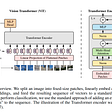## MAE/SimMIM for pre-training like a masked language model